10/19/19 at 19:12:49 News: Registration for new users has been disabled to discourage spam. If you would like to join the forum please send me an email with your desired screen name to tony at gravitysimulator dot com.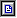Pages: 1 2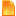faster and better method of integration (Read 15801 times)
 Tony YaBB Administrator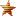Posts: 1060 Gender: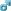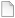Re: faster and better method of integration Reply #15 - 05/28/15 at 20:28:25   I'm busy with 2 graduations this weekend, so probably won't get a chance to look at this until Monday.  Looks good!  Thanks! Back to top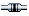IP Logged
 wil UploaderI Love YaBB 2! Posts: 41Re: faster and better method of integration Reply #16 - 05/31/15 at 08:49:34   Quote from frankuitaalst on 05/24/15 at 04:53:52: I mean : introducing higher order in the integration may allow to set larger timesteps for a given "accurancy" . A simple test may be performed : set up a system with central mass and a test particle . The particle should stay within ...x AU after  T time at a timestep of dt . Anxious to see how the integrator performs in GravSim ... I personally use sometimes a 21-order Taylor integrator when I want accurate simulations with self adapting time step .   I tested this one-body verion with circular orbit, and there are some general results:   I just set the 2-body sys. with parameters: y.m = 1; y.r.x = 0; y.v.y = 0; // the central mas, which stays only y.m = 0; y.r.x = 1; y.v.y = 1; // a null particle   The parameters of the orbit are: e = 0, a = 1, GM = 1, then a period is just: T = 2pi (m/a^3)^0.5 = 2pi * 1 = 2pi and a speed: v = 2pi/T = 1   The error is measured as: er = r - 1 = (x^2+y^2)^0.5 - 1   And it is about 1e-9 - 1e-8 for 10 milions of steps, ie. it's no less for any order.   For 2-order method - it's the standard verlet actually, the error is always rather big: 1e-4 or even more for big steps (below about 100 for one orbit: dt < 2pi/100).   For the 4-order and higher the error is 1e-10, and it grows with a time steadily.   There is some limit for a time step size for any order...   In the extremal case: order 16 it's about 10 steps for orbit... and for 20-thy even 4 is sufficient.   The symplectic method 8-order has similar error, as the 8-order extrapolation, but the symplectic orbit is deformed: eccentricity e, and the semi-axis 'a' changes rather strongly - up to 0.001 even; The extrapolated 8-order version keeps perfectly the parameters of the orbit! And the 10-order extrapolated method is high more precise already, than the symplectic 8-order. ... The 12-order variant (k=6) looks very nice - probably the optimal one, for double precision computations.     The simplectic 8-order code is alsor very simple: Code:void symplectic8() { const double W1 = -0.161582374150097E1; const double W2 = -0.244699182370524E1; const double W3 = -0.716989419708120E-2; const double W4 =  0.244002732616735E1; const double W5 =  0.157739928123617E0; const double W6 =  0.182020630970714E1; const double W7 =  0.104242620869991E1; const double W0 = (1-2*(W1+W2+W3+W4+W5+W6+W7)); const static double d8 = { W7, W6, W5, W4, W3, W2, W1, W0, W1, W2, W3, W4, W5, W6, W7 }; const static double c8 = { W7/2, (W7+W6)/2, (W6+W5)/2, (W5+W4)/2, (W4+W3)/2, (W3+W2)/2, (W2+W1)/2, (W1+W0)/2, (W1+W0)/2, (W2+W1)/2, (W3+W2)/2, (W4+W3)/2, (W5+W4)/2, (W6+W5)/2, (W7+W6)/2,  W7/2 };   for(int i = 0; i < 16; i++)    {  h = dt * c8[i];  for(int j = 0; j < nB; j++)     y[j].r.addm(y[j].v, h);   if( i != 15 ) { force(a, y, nB); h = dt * d8[i];   for(int j = 0; j < nB; j++)   y[j].v.addm(a[j], h); }    } }   but the extrapolated method - even limited to the 8-order, is still much better than this.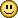Back to topIP Logged
 frankuitaalst Ultimate Member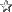Great site Posts: 1510 Gender:Re: faster and better method of integration Reply #17 - 05/31/15 at 11:31:36   Thanks Will for the info and the results !   A question arises to me here :   "The symplectic method 8-order has similar error, as the 8-order extrapolation,    but the symplectic orbit is deformed: eccentricity e, and the semi-axis 'a' changes    rather strongly - up to 0.001 even;    The extrapolated 8-order version keeps perfectly the parameters of the orbit! " The method you describe is very well an extrapolation , not the sympletctic method , or am I wrong ?   In the code here above you seem to mention the symplectic8 . Do I read this well ? Back to topIP Logged
 wil UploaderI Love YaBB 2! Posts: 41Re: faster and better method of integration Reply #18 - 05/31/15 at 16:36:16   Quote from frankuitaalst on 05/31/15 at 11:31:36: The method you describe is very well an extrapolation , not the sympletctic method , or am I wrong ? In the code here above you seem to mention the symplectic8 . Do I read this well ?   This extrapolation is not a symplectic method, it is only based on an symplectic method: the Verlet or Frog.   This schema of integration is type of the RK integration, in fact, but faster and simpler (optimal - minimal in a sense of a number of the forces evaluations per step). A code for, say: RK16 will be tremendously long, complicated, and still slower than the extrapolating schema, which is very simple and compact....   Maybe I compare yet the standard RK4 integrator, with the RKN4... and with a symplectic 4-order.   The code for symplectic8 is a simplectic integrator, of course; this is not the extrapolated: RKN8... which is just realised by a call of: stepk(4);Back to topIP Logged
 wil UploaderI Love YaBB 2! Posts: 41Re: faster and better method of integration Reply #19 - 06/01/15 at 21:21:05   Quote from Tony on 05/23/15 at 13:07:00: Code:         //Apply the Runge-Kutta 4th Order method to these arrays to determine the slope of the position and velocities         //Then add these slopes to the current positions and velocities to get the new positions and velocities.         //Velocity is the derivative of position.         //Acceleration is the derivative of velocity.         //The RK4 method states that the slope for velocity is         // (1/6) * timeStep * (the acceleration at the beginning of the interval, stored in a1x[], a1y[], a1z[]         // + 2 * the acceleration at the first estimate of the half time step, stored in a2x[], a2y[], a2z[]         // + 2 * the acceleration at the second estimate of the half time step, stored in a3x[], a3y[], a3z[]         // + the acceleration at the estimate of the end of a full time step, stored in a4x[], a4y[], a4z[] ).         //The RK4 method states that the slope for position is         // (1/6) * timeStep * (the velocity at the beginning of the interval, stored in v1x[], v1y[], v1z[]         // + 2 * the velocity at the first estimate of the half time step, stored in v2x[], v2y[], v2z[]         // + 2 * the velocity at the second estimate of the half time step, stored in v3x[], v3y[], v3z[]         // + the velocity at the estimate of the end of a full time step, stored in v4x[], v4y[], v4z[] ).         for (k = 1; k <= objects; k++) {            deltavx = (timeStep / 6) * (a1x[k] + 2 * a2x[k] + 2 * a3x[k] + a4x[k]);            deltavy = (timeStep / 6) * (a1y[k] + 2 * a2y[k] + 2 * a3y[k] + a4y[k]);            deltavz = (timeStep / 6) * (a1z[k] + 2 * a2z[k] + 2 * a3z[k] + a4z[k]);              objvx[k] = objvx[k] + deltavx;            objvy[k] = objvy[k] + deltavy;            objvz[k] = objvz[k] + deltavz;         } // Next k         iterations++;     } // Next iteration   } // End function rk4   Do You not lost some part of a code?   I can't see where is a position updated: objx, objy, objz; only a velocity is computed.   probably should be something like this: dr = (h / 6) * (v1 + 2*v2 + 2*v3 + v4);   but: v1 = v v2 = v + a1*h/2 v3 = v + a2*h/2 v4 = v + a3* h   therefore it is just:   dr = h/6 * (v + 2(v + a1*h/2) + 2(v + a2*h/2) + v + a3*h) = h/6 [6v + (a1+a2+a3)h] = hv + h^2/6 (a1+a2+a3)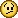Back to topIP Logged
 Tony YaBB AdministratorPosts: 1060 Gender:Re: faster and better method of integration Reply #20 - 06/01/15 at 21:57:15   Sorry, you're correct.  This forum is set to 10k character per post, so I had to break it up into 2 posts, and I must have made a copy and paste error.  Below is the code including position update. Check your private forum inbox tomorrow.  I will send you a copy of the browser version of Gravity Simulator so you can try your code.  It's basically an updated version of the simulations in the "New Gravity Simulator" thread, except this one runs MUCH faster and has a lot more features. Code:         //The RK4 method states that the slope for position is         // (1/6) * timeStep * (the velocity at the beginning of the interval, stored in v1x[], v1y[], v1z[]         // + 2 * the velocity at the first estimate of the half time step, stored in v2x[], v2y[], v2z[]         // + 2 * the velocity at the second estimate of the half time step, stored in v3x[], v3y[], v3z[]         // + the velocity at the estimate of the end of a full time step, stored in v4x[], v4y[], v4z[] ).         for (k = 1; k <= objects; k++) {            deltavx = (timeStep / 6) * (a1x[k] + 2 * a2x[k] + 2 * a3x[k] + a4x[k]);            deltavy = (timeStep / 6) * (a1y[k] + 2 * a2y[k] + 2 * a3y[k] + a4y[k]);            deltavz = (timeStep / 6) * (a1z[k] + 2 * a2z[k] + 2 * a3z[k] + a4z[k]);              objvx[k] = objvx[k] + deltavx;            objvy[k] = objvy[k] + deltavy;            objvz[k] = objvz[k] + deltavz;              deltapx = (timeStep / 6) * (v1x[k] + 2 * v2x[k] + 2 * v3x[k] + v4x[k]);            deltapy = (timeStep / 6) * (v1y[k] + 2 * v2y[k] + 2 * v3y[k] + v4y[k]);            deltapz = (timeStep / 6) * (v1z[k] + 2 * v2z[k] + 2 * v3z[k] + v4z[k]);              objx[k] = objx[k] + deltapx;            objy[k] = objy[k] + deltapy;            objz[k] = objz[k] + deltapz;         } // Next k         iterations++;     } // Next iteration   } // End function rk4 Back to topIP LoggedPages: 1 2